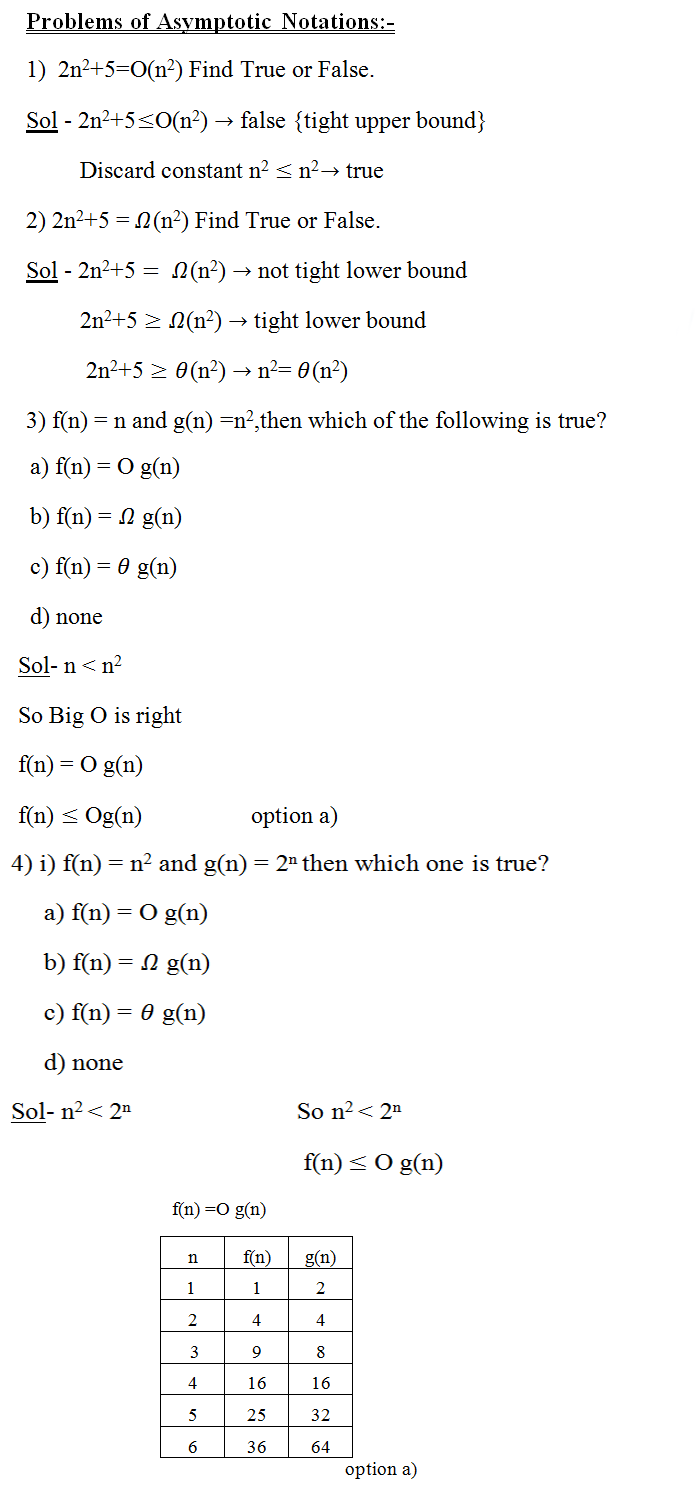## DESIGN AND ANALYSIS OF ALGORITHM

Amortized Algorithm continue and Problems based on Asymptotic Notation
8 November, 2016

3) Potential Method:

A potential function for the data structure that’s initially equal to zero and is always non negative. The amortized cost of an operation is actual cost + Change in potential.

∁ˆᵢ=∁ᵢ+ф(Dᵢ)-ф(Dᵢˍ₁)

The potential is associated with the data structure as a whole rather than with specific objects with the data structure.

Potential Method works as follows :-

1) When start with an initial Data Structure D0 on which n operation are performed.

2) For each i = 1,2,………n  we have actual cost Ci and Di be the data structure that results after applying the operation to Data structure Di-1.

3) The amortized cost of each operation is therefore it’s actual cost plus the increase in potential due to the operation.

∑_(i=1)^n▒〖∁ˡᵢ=∑_(i=1)^n▒〖{∁ᵢ+φ(Dᵢ)-φ(Dᵢˍ^1 ) }  〗〗

A.C of the n operation

4) Amortized Cost in upper bound so it’s higher than Actual Cost If amortized cost is high so potential of the D.S  increases vice versa. It’s also depend on the choice of potential function φ.

e.g.  i) stack operation:- empty stack D0=0  {

Stack is never (-)ve

}

φ(D0)≥0

Amortized cost = ?

If the i+n operation on stack containing s objects is a PUSH operation then the potential difference is
φ(Di)- φ(Di-1) = s+1-s
∁ᵢˡ = ∁ᵢ+ φ(Di)- φ(Di-1)
=  1+1
=    2
POP Operation :- Actual cost =1
Amortized cost=?
Potential Difference = φ(D i)- φ(Di-1)
= 0-1
= -1

So Amortized Cost  =   Ci  = 1-1

= 0

Thus the Amortized cost is o(1).so φ(Di) ≥(D0).

The total amortized cost of n operation’s is O(n) and in Upper Bound.These are some examples based on  Asymptotic Notations.